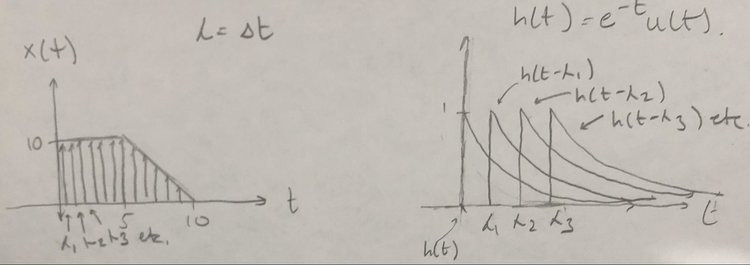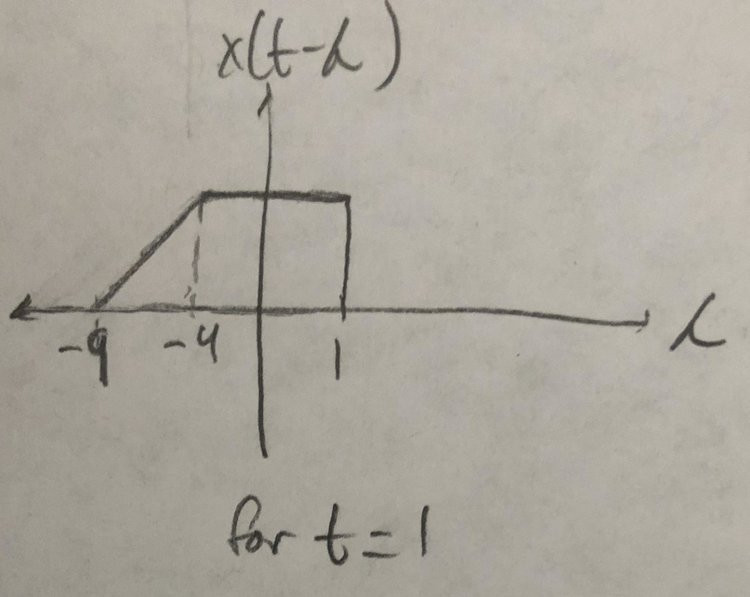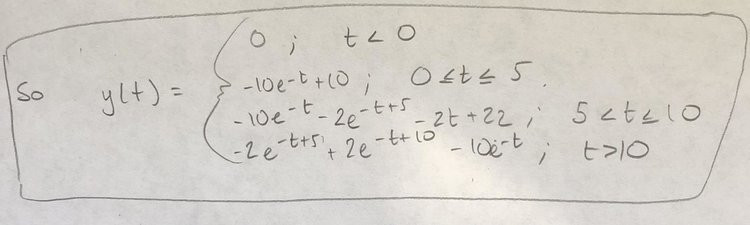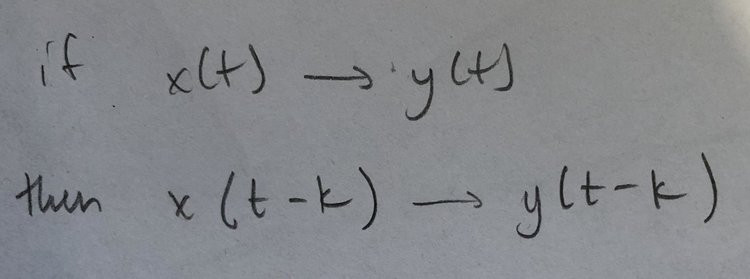# Convolution Interval

Updated: Jan 14, 2020

Calculate the output of this system given that the input signal and the system’s impulse response are:One thing that should be addressed before we start the problem is what is u(t).

u(t) is the step function, or Heaviside step function. It stands for:When multiplied with another function it just means that the function will start at 0, even when it would normally have values below 0. This is why the $$h(t) = e^(-t)u(t)$$ starts at $t = 0$. This also means that if you have an integral from negative infinity to infinity, then it would change to and integral from zero to infinity (since the function equals zero when t = 0)

Next, we should address what convolution even is. Convolution integrals are denoted as y(t) = x(t)*h(t). They can be used to compute the output to a linear, time invariant system with no initial conditions (I’ll go over what those are at the end). Its formula is:So how does convolution actually work?

Basically, you are breaking up the input signal into many individual impulses (lines) at points lambda. You then calculate the output for each one of those lines over time by multiplying the broken up input with the shifted output (shifted by those same values/points, lambda, so the line at lambda 1 is multiplied by the response h(t) shifted to lambda 1). To get the most accurate outcome, we want to have as many of these shifted responses as possible (an infinite amount) added together, so we take the integral of those multiplied components with respect to the shifts (lambda). This represents x(t)*h(t)Note that you could have done the opposite. You could have broken up the impulse response into lines and shifted the input, so h(t)*x(t):We will be using the second way for this problem.

Note: subtracting a constant, like in x(t-k), shifts the graph right, and adding, x(t+k) to the shifts left.

That was the theory, now onto the actual problem. We will use y(t) = h(t)*x(t)

First step is to draw our two graphs, one for h(L), and the other x(t-L) based on the convolution formula. As a heads up, I will be using L for lambda as shorthand.For the graph on the right, the variable being graphed is lambda, so we flip the graph around the y-axis, then we add a constant t, so it gets shifted to the left.

Next step is to consider the intervals we are integrating over. For the sake of solving a convolution integral, we can think of it as a moving integral as you change the constant t (so for instance, if t = 1, then the right edge of x(t-L) will be at 1, the t-5 will be at -4, and the t-10 at -9):The intervals we integrate over is based on where the graph of x(t-L) and h(L) overlap from lambda = negative infinity to infinity. To check where they overlap we change t. So first is t < 0:There is no overlap here, so the output for t < 0 is equal to 0.

Next is partial overlap with the square portion of x(t-L), this will happen from t = 0 to t = 5 (as the square portion ends at [t - 5]):The interval is the shaded region, so lambda = 0 to lambda = t.Next is partial overlap including the slanted section, this happens from t is greater than 5 to t is less than or equal to 10:The interval is still 0 to t, but we have to break it up into two chunks now since the input changes over that interval.The last part is full overlap. This occurs from t is greater than 10 to infinity (so we have satisfied the convolution integral’s definition as an integral from negative infinity to infinity, since we started with t less than 0, which is just from negative infinity to 0, and now we are ending for t greater than 10).. The integration interval is now from t-10 to t, as that’s where the overlap is:Once again we have to break up the input signal x(t-L)That’s the end of the problem. This next part is just a definition for Linear and Time invariant.

Linear:Time Invariant:No initial conditions just means that there is no energy stored before the system starts (so for example in circuits this means that the capacitors and/or inductors don’t have any stored energy/electromagnetic fields).

## Recent Posts

See All## Shayan Raofi

January 14, 2020

View Profile

#### Get in Touch

Thanks! We'll reach out shortly.